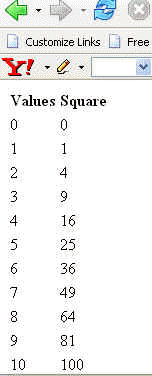Tutorials

# Using JSTL For Finding Square

In this program we are going to find out the square of values from 1 to 10. But the approach we are taking to finding out the square is the JSTL.

In this program we are going to find out the square of values from 1 to 10. But the approach we are taking to finding out the square is the JSTL.

# Using JSTL For Finding Square

In this program we are going to find out the square of values from 1 to 10. But the approach we are taking to finding out the square is the JSTL.

We are using the <c:forEach> core action tag of jstl which will help us to find the square of the values. We are going to find out the square from 1 to 10 which we will give in the attributes of the <c:forEach>. The initial value will be given to attribute var and the last index upto which we have to find out the square will be given in the attribute end.

The code of the program is given below:

 <%@ taglib uri="http://java.sun.com/jstl/core" prefix="c" %>
Values Square

The output of the program is given below: